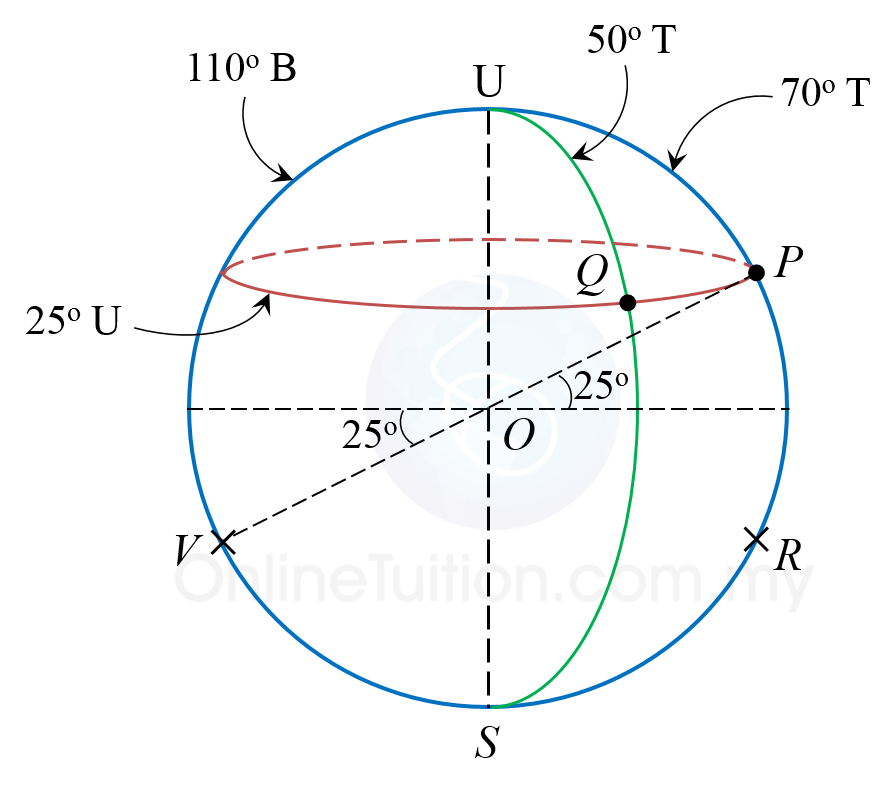# SPM Mathematics 2019, Paper 2 (Question 16)

Question 16:
P(25o U, 70o T). Q(25o U, 50o T). R and F are four points which lie on the surface of the earth. The point R is due south from P. PV is the diameter of the earth.
(a) State the latitude of V.       [2 marks]

(b) Given that the distance of P to R measured along the meridian is 2 580 nautical miles.
Calculate the latitude of R.     [3 marks]

(c) Calculate the shortest distance, in nautical mile, from R to V measured on the surface of the earth.    [2 marks]

(d) An aeroplane took off from Q and flew due east to P and then flew due south to R. The average speed of the aeroplane for the whole flights is 710 knots.
Calculate the total time, in hour, taken for the whole flight.  [5 marks].

Solution:
(a)Latitude of V = 25oS

(b)

(c)
Shortest distance, RV = [(90 – 18) + (90 – 25)]o × 60’
= 137o × 60’
= 8 220 nautical miles

(d)